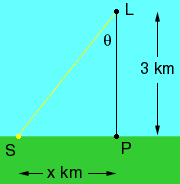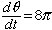melissa student this question is secondary level Q:(involes with related rates) A lighthouse is located on a small island 3 km away from the nearest point P on a straight shoreline, and its light makes 4 revolutions per minute. How fast is the beam of light moving along the shoreline when it is 1 km from P? please help i'm not even sure how to set up the picture for this problem? Hi Melissa, I drew the diagram below. L is the lighthouse, P is the closest point on the shoreline and S is the spot where the light hits the shoreline. L and P are fixed but S moves as the light rotates.I labeled the angle PLS as theta and the changing length |SP| as x. Since the light makes 4 revolutions per minute theta changes 4(2 pi) = 8 pi radians every minute. Henceradians per minute. From the diagram the tangent of theta is  x/3. Can you complete the problem now? Penny Go to Math Central